# Page numbering

The book has 88 pages. How many times is the number 4 used for the book numbering?

Result

n =  19

#### Solution:Leave us a comment of example and its solution (i.e. if it is still somewhat unclear...):

Showing 0 comments:Be the first to comment!#### To solve this verbal math problem are needed these knowledge from mathematics:

See also our permutations calculator. Would you like to compute count of combinations?

## Next similar examples:

1. Book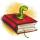Louise read from their favorite book for three days 18 pages. Every day read the same number of pages. How many pages she read every day?
2. Subtraction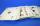How many times you can subtract the number 4 from the number 64?
3. Multiples of int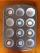How many multiples of 3 is between the numbers to 20? (zero not count)
4. Small painters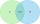There are ten pupils in the painting group. Eight pupils paint with watercolors and nine pupils with tempera colors. How many pupils paint both water and tempera colors when each pupil paints?
5. Unknown number 22I am a two-digit number. My tens digits is 4. My ones digit is 3 more than my tens. Who am I?
6. Mystery numberA mystery number is between 800 and 920. No digits are the same. The difference of the number is 5. What is the mystery number?
7. Parking field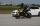There were nine cars in the parking field. The motorcycles were three times less. How many motorcycles are here and how many wheels in the parking field?
8. School marks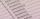Boris has a total of 22 marks. Ones have 3 times less than threes. There are two twos. How many has ones and threes?
9. Mom and dad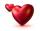Mom wants talk with dad 3 hours. But dad wants of 2 times more hours than mom ... How many hours wants dad talk with mom? ...
10. Three numbers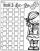How much we increases the sum of three numbers when the first enlarge by 14, second by 15 and third by 16? Choose any three two-digit numbers and prove results.
11. Sum and roundingI know two numbers whose sum is 20. When they each round and then added together I get the 30. What are this numbers?
12. SnacksThe school attends 344 pupils. Half of them take snacks. 13 pupils who took snacks did not attend school. How many snacks left?
13. Cars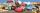Johnny has 370 cars. Peter twice less. How many cars have Peter?
14. Fishing boat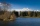The fishing boat caught 14 fish in one day. How many fish will catch 4 fishing boats in 8 days?
15. Car parking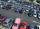The car park has 80 cars when 53 cars leaves. How many car are there?
16. Bus 2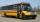On the 6-th stop 44 passengers take off from bus. Overall, the 6-th stop 13 passengers were added. How many passengers take on 6-th stop?
17. The schoolThe school has 268 boys. Girls are 60 more than boys. How many children are there at school?